# The Poisson-binomial distribution for hundreds of parameters

October 7, 2020
By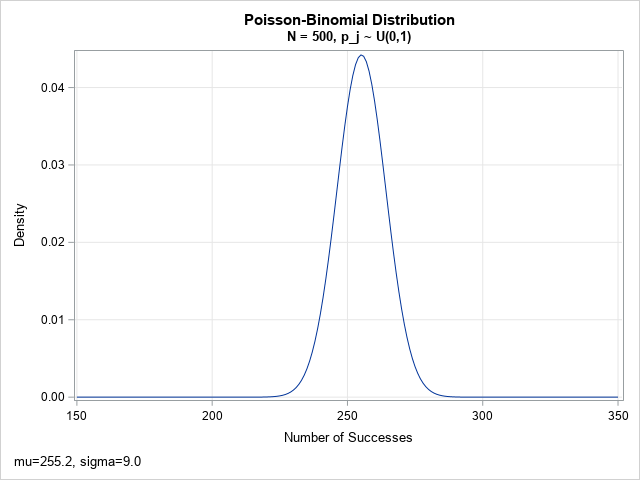A previous article shows how to use a recursive formula to compute exact probabilities for the Poisson-binomial distribution. The recursive formula is an O(N2) computation, where N is the number of parameters for the Poisson-binomial (PB) distribution. If you have a distribution that has hundreds (or even thousands) of parameters,

# Introducing your SAS Global Forum 2021 chair Dina Duhon

October 5, 2020
ByGet acquainted with Dina Duhon, chair of SAS Global Forum 2021.

# Trap and map: Trapping invalid values

October 5, 2020
By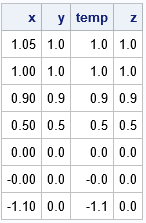Finite-precision computations can be tricky. You might know, mathematically, that a certain result must be non-negative or must be within a certain interval. However, when you actually compute that result on a computer that uses finite-precision, you might observe that the value is slightly negative or slightly outside of the

# How to refactor SAS code to leverage SAS Viya

October 2, 2020
ByIf you're a SAS programmer who now uses SAS Viya and CAS, it's worth your time to optimize your existing programs to take advantage of the new environment. This post is a continuation of my SAS Global Forum 2020 paper Best Practices for Converting SAS® Code to Leverage SAS® Cloud

# Density, CDF, and quantiles for the Poisson-binomial distribution

September 30, 2020
By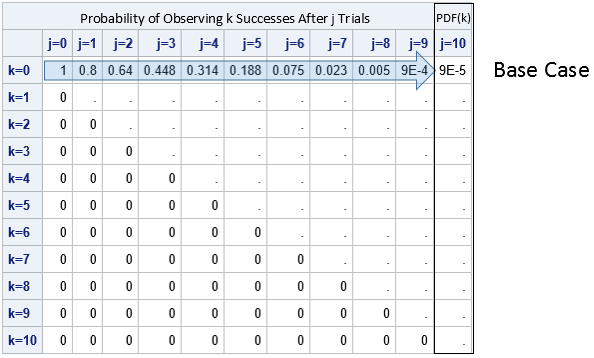When working with a probability distribution, it is useful to know how to compute four essential quantities: a random sample, the density function, the cumulative distribution function (CDF), and quantiles. I recently discussed the Poisson-binomial distribution and showed how to generate a random sample. This article shows how to compute

# The Poisson-binomial distribution

September 28, 2020
By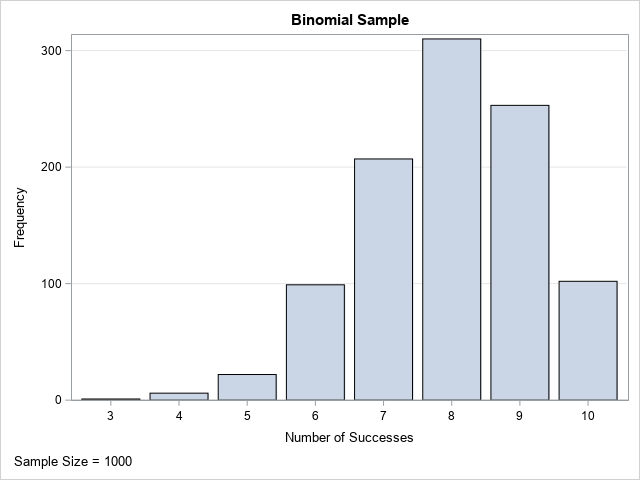The Poisson-binomial distribution is a generalization of the binomial distribution. For the binomial distribution, you carry out N independent and identical Bernoulli trials. Each trial has a probability, p, of success. The total number of successes, which can be between 0 and N, is a binomial random variable. The distribution

# Building custom apps on top of SAS Viya, Part Four: Examples

September 25, 2020
ByWelcome back to my series on securely integrating custom applications into your SAS Viya platform. My goal today is to lay out some examples of the concepts I introduced in the previous posts. As a quick recap: In the first installment of this series I shared my experiences on a

# Working with recurrence relations in SAS

September 23, 2020
By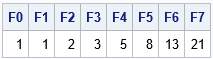Many textbooks and research papers present formulas that involve recurrence relations. Familiar examples include: The factorial function: Set Fact(0)=1 and define Fact(n) = n*Fact(n-1) for n > 0. The Fibonacci numbers: Set Fib(0)=1 and Fib(1)=1 and define Fib(n) = Fib(n-1) + Fib(n-2) for n > 1. The binomial coefficients (combinations

# The value of a low-code/no-code approach to building machine learning models

September 22, 2020
By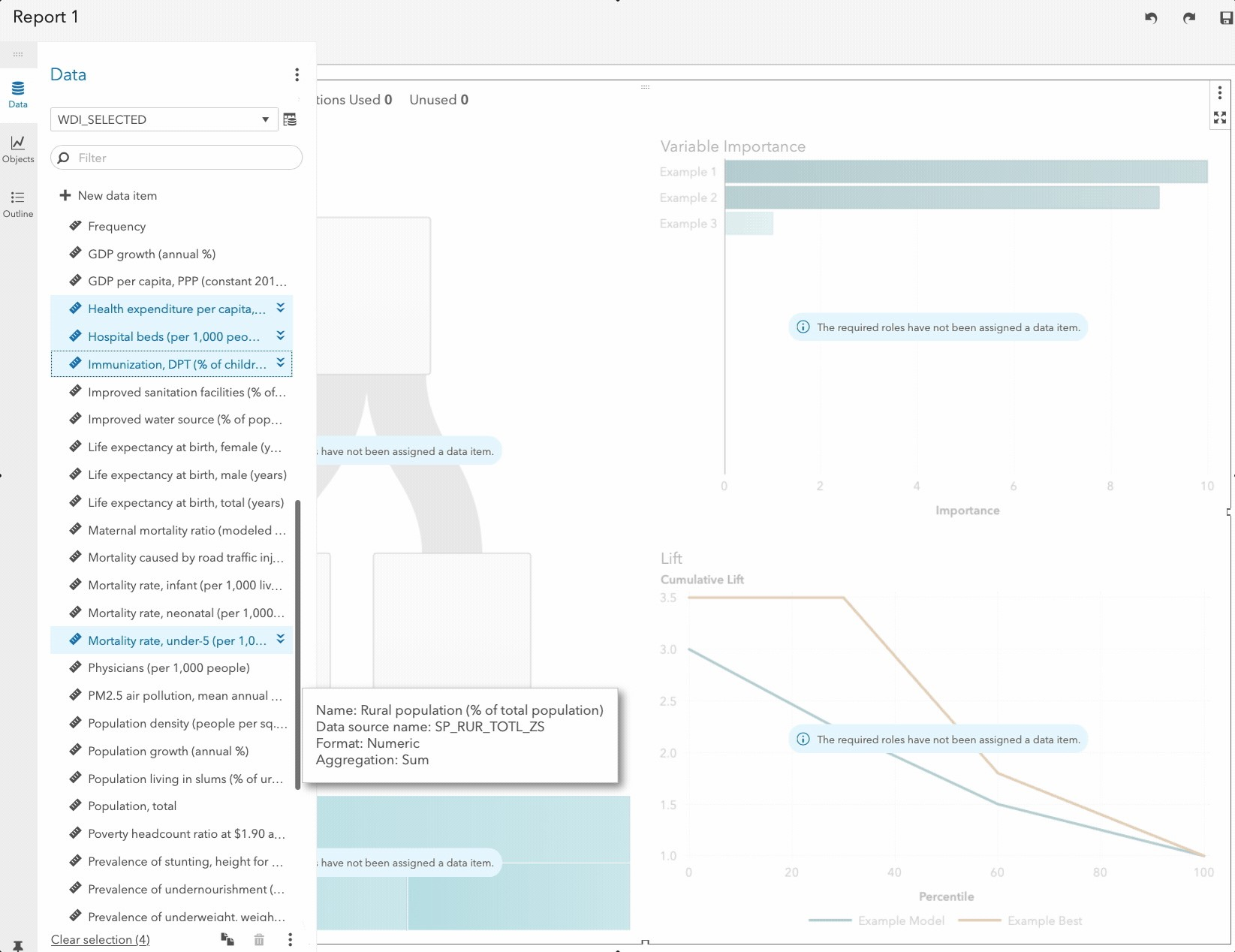Everyone knows that SAS has been helping programmers and coders build complex machine learning models and solve complex business problems for many years, but did you know that you can also now build machines learning models without a single line of code using SAS Viya? SAS has been helping programmers

## Welcome!

SAS-X.com offers news and tutorials about the various SAS® software packages, contributed by bloggers. You are welcome to subscribe to e-mail updates, or add your SAS-blog to the site.## Recent Posts

SAS and all other SAS Institute Inc. product or service names are registered trademarks or trademarks of SAS Institute Inc. in the USA and other countries. ® indicates USA registration.